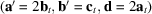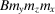International Tables for Crystallography (2006). Vol. E, ch. 5.2, pp. 393-416   | 1 | 2 | https://doi.org/10.1107/97809553602060000652

## Contents

• 5.2. Guide to the use of the scanning tables  (pp. 393-416)
• 5.2.1. Introduction  (pp. 393-394) | html | pdf |
• 5.2.2. The basic concepts of the scanning  (pp. 394-398) | html | pdf |
• 5.2.2.1. The scanning for sectional layer groups  (pp. 394-395) | html | pdf |
• 5.2.2.2. The scanning group and the scanning theorem  (p. 395) | html | pdf |
• 5.2.2.3. The conventional basis of the scanning group  (pp. 395-396) | html | pdf |
• 5.2.2.4. The types of scanning  (p. 396) | html | pdf |
• 5.2.2.5. Orientation orbits  (pp. 396-397) | html | pdf |
• 5.2.2.6. Linear orbits  (pp. 397-398) | html | pdf |
• 5.2.2.7. Orthogonal, inclined and triclinic scanning  (p. 398) | html | pdf |
• 5.2.3. The contents and arrangement of the scanning tables  (pp. 398-402) | html | pdf |
• 5.2.3.1. The standard format  (pp. 398-401) | html | pdf |
• 5.2.3.1.1. Headline  (pp. 398-399) | html | pdf |
• 5.2.3.1.2. Orientation orbit  (p. 399) | html | pdf |
• 5.2.3.1.3. The scanning group and its conventional basis  (pp. 399-400) | html | pdf |
• 5.2.3.1.4. The linear orbits and sectional layer groups  (pp. 400-401) | html | pdf |
• 5.2.3.2. Auxiliary tables  (pp. 401-402) | html | pdf |
• 5.2.3.2.1. Tables of orientation orbits and auxiliary bases of scanning groups  (p. 401) | html | pdf |
• 5.2.3.2.2. Reference tables  (pp. 401-402) | html | pdf |
• 5.2.4. Guidelines for individual systems  (pp. 402-410) | html | pdf |
• 5.2.4.1. Triclinic system  (p. 402) | html | pdf |
• 5.2.4.2. Monoclinic system  (pp. 402-403) | html | pdf |
• 5.2.4.3. Orthorhombic system  (pp. 403-404) | html | pdf |
• 5.2.4.3.1. Orthogonal scanning, standard tables  (p. 403) | html | pdf |
• 5.2.4.3.2. Inclined scanning, auxiliary tables  (pp. 403-404) | html | pdf |
• 5.2.4.4. Tetragonal system  (pp. 404-405) | html | pdf |
• 5.2.4.4.1. Orthogonal scanning, standard tables  (p. 404) | html | pdf |
• 5.2.4.4.2. Inclined scanning, auxiliary tables  (pp. 404-405) | html | pdf |
• 5.2.4.5. Hexagonal family  (pp. 405-407) | html | pdf |
• 5.2.4.5.1. Orthogonal scanning, standard tables  (pp. 405-407) | html | pdf |
• 5.2.4.5.2. Inclined scanning, auxiliary tables  (p. 407) | html | pdf |
• 5.2.4.6. Cubic system  (pp. 407-410) | html | pdf |
• 5.2.4.6.1. Orthogonal scanning, standard tables  (pp. 408-409) | html | pdf |
• 5.2.4.6.2. Inclined scanning, auxiliary tables  (pp. 409-410) | html | pdf |
• 5.2.5. Applications  (pp. 410-415) | html | pdf |
• 5.2.5.1. Layer symmetries in crystal structures  (pp. 410-411) | html | pdf |
• 5.2.5.2. Interfaces in crystalline materials  (pp. 411-412) | html | pdf |
• 5.2.5.3. The symmetry of domain twins and domain walls  (pp. 412-415) | html | pdf |
• References | html | pdf |
• Figures
• Fig. 5.2.2.1. Sets of parallel planes (left) and sets of parallel straight lines (right)  (p. 394) | html | pdf |
• Fig. 5.2.2.2. Monoclinic/orthogonal (left) and monoclinic/inclined (right) scanning  (p. 398) | html | pdf |
• Fig. 5.2.4.1. Six monoclinic cell choices  (p. 402) | html | pdf |
• Fig. 5.2.4.2. Symbols for a hexagonal lattice with a rectangular point group  (p. 405) | html | pdf |
• Fig. 5.2.4.3. Another choice of orthogonal basis vectors for a hexagonal lattice  (p. 405) | html | pdf |
• Fig. 5.2.4.4. The relationship between hexagonal and rhombohedral bases in the obverse setting  (p. 406) | html | pdf |
• Fig. 5.2.4.5. The diagram of the scanning groupin the plane of orientationprojected orthogonally along(p. 406) | html | pdf |
• Fig. 5.2.4.6. The diagram of the scanning groupin the plane of orientationprojected orthogonally along(p. 406) | html | pdf |
• Fig. 5.2.4.7. Illustration of the transformation of Bravais–Miller indices in a hexagonal basis to Bravais indices in an auxiliary basis  (p. 407) | html | pdf |
• Fig. 5.2.4.8. Vectors along the main cubic axes  (p. 408) | html | pdf |
• Fig. 5.2.4.9. The cubic scanning for orientation (111) in the case of cubic groups with a P lattice  (p. 408) | html | pdf |
• Fig. 5.2.4.10. The cubic scanning for orientation (111) in the case of cubic groups with an I lattice  (p. 409) | html | pdf |
• Fig. 5.2.4.11. The cubic scanning for orientation (111) in the case of cubic groups with an F lattice  (p. 409) | html | pdf |
• Fig. 5.2.5.1. The structure of cadmium iodide, CdI 2   (p. 410) | html | pdf |
• Fig. 5.2.5.2. The structure of cadmium chloride, CdCl 2   (p. 411) | html | pdf |
• Fig. 5.2.5.3. A classical example of a bicrystal (Vlachavas, 1985)  (p. 411) | html | pdf |
• Fig. 5.2.5.4. The four types of operations on a twin  (p. 412) | html | pdf |
• Fig. 5.2.5.5. The unit cell of the parent structure of calomel and the cells of four ferroic domain states  (p. 413) | html | pdf |
• Fig. 5.2.5.6. The unordered domain pair between the two domain states  (p. 414) | html | pdf |
• Fig. 5.2.5.7. The structures and symmetries of domain twins in calomel corresponding to two different special positions of the wall  (p. 415) | html | pdf |
• Tables
• Table 5.2.2.1. Various types of scanning  (p. 396) | html | pdf |
• Table 5.2.5.1. Symmetries of domain states and domain pairs in a calomel crystal  (p. 414) | html | pdf |
• Table 5.2.5.2. Sectional layer groups of space groupsandin the conventional basisof the scanning groupand the respective twin symmetries  (p. 414) | html | pdf |